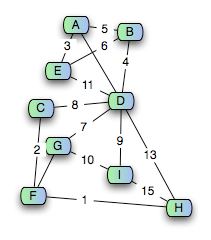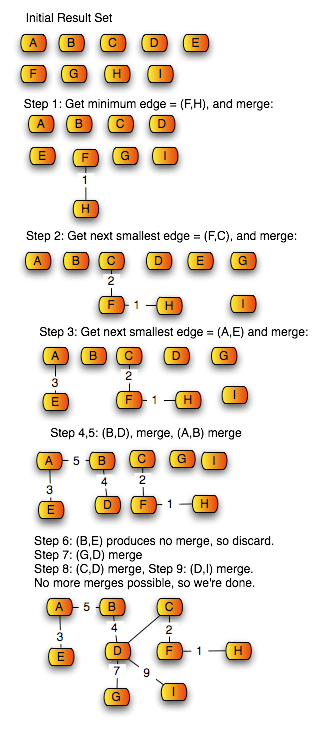# Weighted Graphs and the Minimum Spanning Tree

Moving on from simple graphs, one of the things you can do to make things more interesting
is to associate numeric values with the edges, called the weights of those edges. This variation
of a graph is called a weighted graph.

Weighted graphs are extremely useful buggers: many real-world optimization problems ultimately
reduce to some kind of weighted graph problem. A few examples include:

• The traveling salesman problem (TSP): given a set of cities, and information about travel-times
between pairs of cities, find the shortest route that visits all cities. This is actually an
incredibly commonly used problem; for example, in large data centers which manage backups,
a set of backup-restore requests is served by computing the seek time between pairs
of requests (where seek time includes finding the correct tape, loading it into a drive, and
scanning to the correct point on tape), and then finding the optimum order in which to fulfill
the requests by using a TSP algorithm
• Shortest path problem: a subset of the TSP; given a set of cities and information about
travel-time between cities with direct transportation routes, find the shortest path between
two cities.
• The minimum spanning tree problem: given a weighted graph, find the spanning tree
with minimum total edge-weight. Airlines use minimum spanning trees to work out their basic
route system: flights between hubs are low-cost; other flights have varying prices depending on how many people fly them, what airplanes the airports can support, fuel transport costs, etc. The best
set of routes is the minimum spanning tree.

Formally, a weighted graph is defined by a triple: (V, E, W), where:

• V is a set of vertices;
• E is a set of edges {v,w} where v,w∈V.
• W is a map from edges to numbers. (Depending on the specifics of a problem,
the numbers might be restricted to positive numbers, natural numbers,
integers, …)

For today, we’ll look at the last of those in a bit more detail: finding the minimum spanning tree. This is one of the problems that constantly comes up in infrastructure design – from building layouts, to
network structure to warehouse location. One great example of the use of this is in telecommunications. When AT&T and Sprint were laying fiber optic cable to replace their copper-wire backbone, they
took their primary routing stations, and arranged them in a graph. Then they worked out the cost of
connecting each pair of routing stations by fiber, and applied those costs as edge-weights. Then they
computed the minimum spanning tree of the resulting graph – and that defined the least-expensive
way to lay the fiber for a new backbone.

So let’s start by making the definition of the minimum spanning tree problem more precise.

1. Suppose we have a simple graph, G=(V,E). A spanning tree SG= (V,E’) is a subtree (acyclic subgraph) of G, where E’⊆E.
2. If G is a weighted graph G=(V,E,W), then the weight of G = Σe∈EW(e) – that is, the sum of the weights of all of the edges in the graph.
3. If G is a weighted graph, then the minimum spanning tree Span(G) is the spanning tree over
G with minimum weight.

Given that just about every interesting graph problem that we’ve seen has turned out to be NP-complete, you might expect finding a minimum spanning tree to be NP-complete as well. But graphs are often counterintuitive: things that seem like they should be simple turn out to be very difficult; things that look like they’ll be difficult sometimes turn out to be simple. In fact, there’s a very simple algorithm which is O(n lg n) where n is the number of edges.

It’s called Kruskal’s algorithm. And it’s a really beautiful algorithm – an extraordinarily clear,
simple, easy way of solving the problem. It’s based on what’s known as a greedy approach. In a
greedy approach, you aggressively grab the thing that looks best, try it out, and see if it fits.

In Kruskal’s algorithm, you greedily grab edges – you always grab the lowest weight edge, and see if it
helps build a spanning tree; if so, you add it, if not you discard it; and you keep doing that until
you have the spanning tree. (From that description, you might think that it’s really O(N) in the number of edges – because you consider each edge once. But the edges have to be in sorted order, and sorting the edges is O(N lg N).)

So here’s the algorithm more precisely:

```FindMST(G=(V,E,W))
let Result = a set of trees, each consisting of one node from G
let SortedEdges = sort(E,W)
for each MinEdge in SortedEdges:
if MinEdge connects two disconnected trees in Result:
merge the trees in Result by adding MinEdge
else:
end if
end for
```

Let’s walk through an example. Here’s a graph with weighted edges:Now we’ll go through Kruskal’s algorithm to generate the MST:So the final weight of the minimum spanning tree for this graph is 31.

## 0 thoughts on “Weighted Graphs and the Minimum Spanning Tree”

1.Maya Incaand

Don’t forget Prim (Jarnik) and Boruvka.
Wonder why the old East bloc is so big on algorithms.

2.Torbjörn Larsson, OM

FWIW, since this blog so often has occasion to criticize the bad math of creationists, I was curious to see if not spanning trees pops up in biology.
Googling indicates that it does, in descriptions of populations development (evolutionary and/or biogeographically). Cladistics seems to be one obvious application, where for example the descent tree (on population characteristics) with most bayesian likelihood can be searched for.

3.Jon Olson

The important bit to remember with Kruskal’s is that you have to be careful about the data structure you use to represent connected trees. If you’re having to check for connectivity between trees every time your algorithm is O(|E|2) (I might have to check every edge for each new edge I add). With Union-Find you get that down to O(N log* N) over the run of your algorithm, thus allowing sorting to dominate the running time.
It is for this reason that would tend to recommend Prim’s algorithm to people who aren’t picky since it has (worst case using and adjacency matrix to search for the lowest weight weight edge connected to the tree) time complexity O(|V|2).

4.ugstu

Airlines use minimum spanning trees to work out their basic route system

When AT&T and Sprint were laying fiber optic cable to replace their copper-wire backbone, […] they computed the minimum spanning tree of the resulting graph – and that defined the least-expensive way to lay the fiber for a new backbone.

Guess I have very little intuition for the real world 🙂
I don’t understand why this should be so — while it’s obvious that the strictly least-expensive way is a tree, wouldn’t it make sense to directly connect some far-apart but often-used airports, if the distance between them (according to the MST) is high? And similarly with the communications network…
Perhaps the answer is that because the graph is actually laid out on a plane and costs are roughly proportional to the Euclidean distance, the “direct” edge between two vertices won’t be so much cheaper than the distance given by the MST that it’s worth adding the extra (redundant) edge. Is this correct?
Still I find it hard to believe that MSTs would be used… what if one crucial node went down?

5.Skemono

Keep in mind, ugstu, that the “weight” of an edge may not be as simple as the distance between the nodes. It could be derived from a complicated algorithm that takes into account distance, money, frequency of use, and all sorts of things.

6.Mark C. Chu-Carroll

ugstu:

what if one crucial node went down?

Remember the JetBlue chaos this past winter at JFK when it snowed? JFK is one of JetBlue’s hubs – the major branch-point
in their tree.
Most airlines have adopted what they call a hub-and-spoke
routing system. That means that they have a relatively small number of major airports that they work out of, and most of their flights are routed primarily through the hubs. For example, my family is going on vacation to Yellowstone this summer. The only way we can get there is by taking a flight from Newark to Chicago, and then Chicago to some dinky airport near the park. Chicago and Newark are Continental hubs.
The airline’s full route isn’t a tree – they do augment the route with extra edges between hubs. But the basic routing is an MST, with manual tweaks afterwards.

7.Fred

How is this different than Linear Programing as described by George Danzig? As to the objective function being non-linear, that is called in industry as parametric Linear Programming . How is your algorithm different than a simplex?

8.prabhat kumar

pls mail me collection on weighted shortest graph.